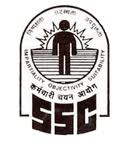### Syllabus of SSC CGL TIER-II Examination 2013

#### About SSC CGL Recruitment 2013One of the most popular Government exams- SSC's Combined Graduate Level (CGL) 2013 Notification is Out and Online Registrations are going on, The Last Date to Submit the SSC CGL Online form is 15th February, 2013. and Written Exam is Scheduled on 14th and 21st April, 2013. Commission will be holding Combined Graduate Level Examination,2013 for recruitment to different posts for which Graduation from a recognized University is the minimum Educational Qualification. The Examination will comprise of two Tiers of Written Objective Type examination followed by Computer Proficiency Test/ Interview/Skill test, wherever applicable as per the Scheme of Examination and a descriptive/on-line test of qualifying nature for candidates shortlisted for interview/skill test/document verification.

#### About Syllabus of SSC CGL TIER-II Examination

Paper-I : Quantitative Ability - 200 Marks-100 Questions: The questions will be designed to test the ability of appropriate use of numbers and number sense of the candidate. The scope of the test will be the computation of whole numbers, decimals ,fractions and relationships between numbers, Percentage. Ratio & Proportion,Square roots, Averages, Interest, Profit and Loss, Discount, Partnership Business, Mixture and Alligation, Time and distance, Time & Work, Basic algebraic identities of School Algebra & Elementary surds, Graphs of Linear Equations, Triangle and its various kinds of centres, Congruence and similarity of triangles, Circle and its chords, tangents, angles subtended by chords of a circle, common tangents to two or more circles, Triangle, Quadrilaterals, Regular Polygons , Circle, Right Prism, Right Circular Cone, Right Circular Cylinder, Sphere, Hemispheres, Rectangular Parallelepiped, Regular Right Pyramid with triangular or square base, Trigonometric ratio, Degree and Radian Measures, Standard Identities, Complementary angles, Heights and Distances, Histogram, Frequency polygon, Bar diagram & Pie chart.

Paper-II : English Language & Comprehsion - 200 Marks-200 Questions :Questions in this components will be designed to test the candidate’s understanding and knowledge of English Language and will be based on spot the error, fill in the blanks, synonyms, antonyms, spelling/detecting mis-spelt words, idioms & phrases, one word substitution, improvement of sentences, active/passive voice of verbs, conversion into direct/indirect narration, shuffling of sentence parts, shuffling of sentences in a passage, cloze passage & comprehension passage

Paper-III : -200 Marks-100 Questions- Statistics for Investigator Grade-II, Ministry of Statistics & Programme Implementation & Compiler in RGI. Collection Classification and Presentation of Statistical Data – Primary and Secondary data, Methods of data collection; Tabulation of data; Graphs and charts; Frequency distributions; Diagrammatic presentation of frequency distributions. Measures of Central Tendency- Common measures of central tendency – mean median and mode; Partition values- quartiles, deciles, percentiles. Measures of Dispersion- Common measures dispersion – range, quartile deviations, mean deviation and standard deviation; Measures of relative dispersion. Moments, Skewness and Kurtosis – Different types of moments and their relationship; meaning of skewness and kurtosis; different measures of skewness and kurtosis. Correlation and Regression – Scatter diagram; simple correlation coefficient; simple regression lines; Spearman’s rank correlation; Measures of association of attributes; Multiple regression;
Multiple and partial correlation (For three variables only). Probability Theory – Meaning of probability; Different definitions of probability; Conditional probability; Compound probability; Independent events; Bayes’ theorem. Random Variable and Probability Distributions – Random variable; Probability functions; Expectation and Variance of a random variable; Higher moments of a random variable; Binomial , Poisson, Normal and Exponential distributions; Joint distribution of two random variable (discrete). Sampling Theory – Concept of population and sample; Parameter and statistic, Sampling and non-sampling errors; Probability and non-probability sampling techniques(simple random sampling, stratified sampling, multistage sampling, multiphase sampling, cluster sampling, systematic sampling, purposive sampling, convenience sampling and quota sampling); Sampling distribution(statement only); Sample size decisions. Statistical Inference - Point estimation and interval estimation, Properties of a good estimator,
Methods of estimation (Moments method, Maximum likelihood method, Least squares method), Testing of hypothesis, Basic concept of testing, Small sample and large sample tests, Tests based on Z, t, Chi-square and F statistic, Confidence intervals. Analysis of Variance - Analysis of one-way classified data and two-way classified data. Time Series Analysis - Components of time series, Determinations of trend component by different methods, Measurement of seasonal variation by different methods. Index Numbers - Meaning of Index Numbers, Problems in the construction of index numbers, Types of index number, Different formulae, Base shifting and splicing of index numbers, Cost of living Index Numbers, Uses of Index Number.

NOTE-I : Questions in Paper-I will be of 10 th standard level, Paper-II of 10+2 level and Paper-III of graduation level.

NOTE- II: (i)The Commission will have full discretion to fix separate minimum qualifying marks in each of the papers in Tier II and in the aggregate of all the papers separately for each category of candidates (viz. SC/ST/OBC /PH/ExS/General (UR) ). Only those candidates who qualify in all the papers as well as in the aggregate would be eligible to be considered for being called for Interview and/or Skill Test. (ii)There will be different set of Questions for Visually Handicapped (VH) candidates in Paper-I-Quantitative Ability, which shall not have any component of Map/Graphs/Statistical Data/ Diagrams/Figures/Geometrical problems/Pie-chart etc. However, components of other papers will be the same as that for general candidates.

NOTE-III: Any representation to key of the examination will be scrutinized with the help of experts, wherever necessary, and evaluation in that case will be done with modified answer key. The decision of the Commission in this regard will be final and no further representation will be entertained.